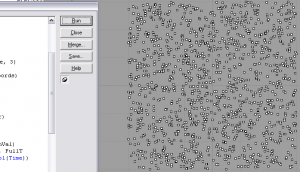Skimming thru my copy of “Algorithms in C” by Sedgewick, I stumbled upon the Linear Congruence method of simulating randomness.  I was able to convert it to Rhinoscript quite easily.  It’s a method of pseudo-randomness that uses the modulus to extract remainders.  Since most remainders are somewhat random (or as Sedgewick calls it, “arbitrary”) it is good enough for simulating naturally random numbers.  It was first introduced to computer programming by D. Lehmer in 1951.

The example in the book (in C) uses a syntax that reuses the constants from the previous calculation.  In the Rhinoscript version I wrote below, I used RValue, ConstM and ConstB as variants.  In newer version (not listed), I’ve replaced the static ConstB value with the assignment = (CDbl(Time)*1000), so that each iteration is different.  Otherwise, your number sequence is identical each time.  Either way will work for you, especially if you are looking to capture all the random outputs into a matrix or txt file.  But that’s up to you.

Note, something not shown in this working function is the public variable declaration of the RValue.  You’ll need that defined so that each new iteration of the sequence, it references the new random number.  For those of you new to programming, this is a classic example of a recursive sequence, which I’ve gone over in depth in my book YSYT, on pages 68 to 84.

I threw the function on the wiki:

http://www.nickpisca.com/BLAST/index.php?title=RVB_LinCong

```Public Function LinCong()
Dim OT, ConstB, ConstM
ConstB = 4.1
ConstM = 100
OT = ((RValue*ConstB)+1) Mod ConstM
RValue = OT
LinCong = OT
End FunctionNick Pisca's rhinoscript lin cong algorithm.```